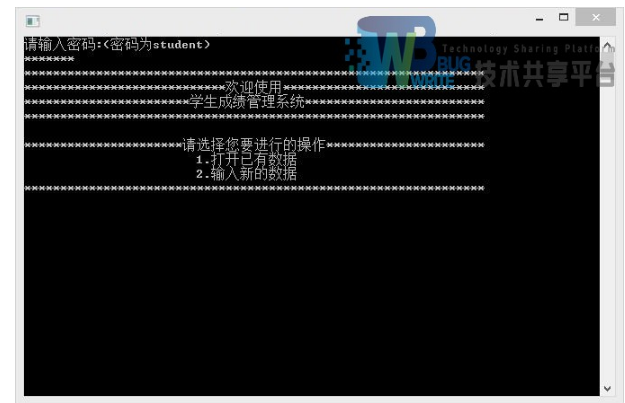• 假设收集到了一个班学生的所有成绩信息(不低于20人)，要求用C语言编写一个简单的成绩管理系统，对学生个人信息进行管理，可进行录入、浏览、查询、修改、排序、统计等功能。信息内荣包括：(1)学生的学号(2)学生的...
C语言实现一个简单的成绩管理系统
实验内容
假设收集到了一个班学生的所有成绩信息(不低于20人)，要求用C语言编写一个简单的成绩管理系统，对学生个人信息进行管理，可进行录入、浏览、查询、修改、排序、统计等功能。信息内荣包括：(1)学生的学号(2)学生的姓名(3)学生的成绩(语文、数学、英语、计算机、课程平均分)。
1.录入、浏览功能：
按学号顺序录入学生信息(学号、姓名、语文、数学、英语、计算机)显示系统中的所有信息。
2.查询功能：
查询学号尾号为09的学生的所有信息并显示。
3.修改功能：
修改学号尾号为12的学生的成绩信息(任意两项即可)，并显示该学生的全部信息。
4.排序功能：
按语文成绩的顺序，由高到低显示所有学生的信息。
5.统计功能：统计学生的平均成绩，并输出(学号、姓名、平均成绩)
#include<stdio.h>
#include<stdlib.h>
int n=5;
int no;
struct stu
{
char name;
int num;
int chinese;
int math;
int english;
int computer;
float average;
}student;
//1.输入信息
void student_scanf(int n)
{
for(int i=0;i<n;i++)
{	printf("请输入第%d个学生的信息：\n",i+1);
printf("学号  姓名  语文成绩  数学成绩 英语成绩  计算机成绩\n");
scanf("%d%s%d%d%d%d",&student[i].num,&student[i].name,&student[i].chinese,&student[i].math,&student[i].english,&student[i].computer);
student[i].average=(student[i].chinese+student[i].math+student[i].english+student[i].computer)/4.0;
}
}
void student_printf(int n)
{
printf("学号  姓名  语文成绩  数学成绩  英语成绩  计算机成绩  平均成绩\n");
for(int i=0;i<n;i++)
{
printf("%d\t%s\t%d\t%d\t%d\t%d\t%f\n",student[i].num,student[i].name,student[i].chinese,student[i].math,student[i].english,student[i].computer,student[i].average);
}
}

void student_seek(int no)
{
int j=0;
for(int i=0;i<n;i++)
{
if (no==student[i].num)
{
j=1;
printf("学号：%d 姓名：%s 语文：%d 数学：%d 英语：%d计算机：%d 平均成绩；%f\n",student[i].num,
student[i].name,student[i].chinese,student[i].math,student[i].english,student[i].computer,student[i].average);
break;
}
}
if(j==0) printf("查询的学号不存在！\n");
}
void student_alter(int no)
{

for(int i=0;i<n;i++)
{
if (no==student[i].num)
{

printf("姓名：");
scanf("%s",&student[i].name);
printf("语文成绩：");
scanf("%d",&student[i].chinese);
printf("数学成绩：");
scanf("%d",&student[i].math);
printf("英语成绩：");
scanf("%d",&student[i].english);
printf("计算机成绩：");
scanf("%d",&student[i].computer);
student[i].average=(student[i].chinese+student[i].math+student[i].english+student[i].computer)/4.0;
printf("修改后的信息为：");
printf("%d\t%s\t%d\t%d\t%d\t%d\t%f\n",student[i].num,student[i].name,student[i].chinese,
student[i].math,student[i].english,student[i].computer,student[i].average);
}
}
}
void student_sort_chinese()
{
for (int i = 0; i < n - 1; i++)
{
for (int j = 0; j < n - i - 1; j++)
{
if (student[j].chinese < student[j + 1].chinese)
{
stu temp = student[j];
student[j] = student[j + 1];
student[j + 1] = temp;
}

}
}

}
void Statistics()
{
printf("学号\t姓名\t平均成绩\n");
for(int i=0;i<n;i++)
{
printf("%d\t%s\t%f\n",student[i].num,student[i].name,student[i].average);
}

}
int main(){
student_scanf(n);
student_printf(n);
printf("请输入要查询的学生的学号：");
scanf("%d",&no);
student_seek(no);
printf("请输入修改信息的学生的学号");
scanf("%d",&no);
student_alter(no);
printf("按语文成绩从大到小排序：\n");
student_sort_chinese() ;
student_printf(n);
printf("统计信息为：\n");
Statistics();
return 0;
}



展开全文• 计算机技术基础课程设计 C语言 设计报告 题目学生成绩管理系统 选题背景 问题的提出在学校的学生管理工作过程中对学生的成绩统计管理是一个 非常必要的问题就此编写此C程序对一个班的学生成绩进行管理其中每 ...
• 编写一个学生成绩管理系统，实现对班级学生成绩的录入、修改、查找、删除、显示、排序、统计、保存等操作管理。二 程序设计2.1 功能设计系统功能如下：录入每个同学学号、姓名、性别和各科考试成绩成绩修改：...
一 需求分析假设某班有不超过40人(具体人数在程序运行时由键盘录入)参加本学期的期末考试，考试科目为语文(CN)，数学(MT)，英语(EN)，科学(SC)。编写一个学生成绩管理系统，实现对班级学生成绩的录入、修改、查找、删除、显示、排序、统计、保存等操作的管理。二 程序设计2.1 功能设计系统功能如下：录入每个同学的学号、姓名、性别和各科的考试成绩成绩修改：可以根据输入的学号修改成绩；在修改之前需要用户进一步确认，确认无误后再进行操作计算每门课程的总分和平均分计算每个学生所有课程的总分和平均分按每个学生的总分进行排序按学号排出成绩表按姓名的字典顺序排出成绩表按姓名查询学生的各科成绩、总分、平均分及在班级的排名按学号查询学生的各科成绩、总分、平均分及在班级的排名对每门课程按优(90~100)、良(80~89)、中(70~79)、及格(60~69)、不及格(0~59)统计人数，及所占的百分比输出每个学生的姓名、学号、性别、各门课程的考试成绩、总分、平均分及在班级内的排名输出班级每门课程的统计数据：包括每门课程的平均分、每门课程优、良、中、及格、不及格的人数及所占百分比将每个学生的相关信息写入文件保存从文件中读出相关信息并进行处理退出系统参考文档和完整的文档和源码下载地址：
展开全文• Problem Description 转眼又到了一年年末，Lele又次迎来了期末考试。...对于每组数据，请在行里输出里一共有多少个学生成绩高于Lele Sample Input 1 3 2 81 72 63 Sample Output 1Golang erlang r语言
• 假设一个班有n(n)个学生，每人考m(m)门课，求每个学生的平均成绩和每门课的平均成绩，并输出各科成绩均大于等于平均成绩的学生数量。 Input 输入数据有多个测试实例，每个测试实例的第一行包括两个整数n和m，...Golang erlang r语言
• 编写一个学生成绩管理系统，实现对班级学生成绩的录入、修改、查找、删除、显示、排序、统计、保存等操作管理。 二 程序设计 2.1 功能设计 系统功能如下： 录入每个同学学号、姓名、性别和各科考试成绩 ...
一 需求分析

假设某班有不超过40人（具体人数在程序运行时由键盘录入）参加本学期的期末考试，考试科目为语文（CN），数学（MT），英语（EN），科学（SC）。编写一个学生成绩管理系统，实现对班级学生成绩的录入、修改、查找、删除、显示、排序、统计、保存等操作的管理。

二 程序设计

2.1 功能设计

系统功能如下：

录入每个同学的学号、姓名、性别和各科的考试成绩

成绩修改：可以根据输入的学号修改成绩；在修改之前需要用户进一步确认，确认无误后再进行操作

计算每门课程的总分和平均分

计算每个学生所有课程的总分和平均分

按每个学生的总分进行排序

按学号排出成绩表

按姓名的字典顺序排出成绩表

按姓名查询学生的各科成绩、总分、平均分及在班级的排名

按学号查询学生的各科成绩、总分、平均分及在班级的排名

对每门课程按优（90~100）、良（80~89）、中（70~79）、及格（60~69）、不及格（0~59）统计人数，及所占的百分比

输出每个学生的姓名、学号、性别、各门课程的考试成绩、总分、平均分及在班级内的排名

输出班级每门课程的统计数据：包括每门课程的平均分、每门课程优、良、中、及格、不及格的人数及所占百分比

将每个学生的相关信息写入文件保存

从文件中读出相关信息并进行处理

退出系统点击此处下载文档和源码


展开全文• 程序使用说明：本程序是使用C语言编写的学生成绩管理系统，使用VS2013进行代码的编写，其中file1.dat是实验数据，file2.dat是 排序后的数据。 实习题目：学生成绩管理系统 要求： 1、增加记录：要求可以连续增加...
• 一个学生成绩管理系统 学生成绩有语文 数学 英语 功能 录入成绩 找三科总分最高分 最低分 算出平均分 学生结构体 struct Student { int Stunum; //学号 int Chinese; int Math; int English; int Sum; //...
场景
一个年级，相当链表A，该年级5个班，每个班5个人，相当于链表B1–B5
做一个学生成绩管理系统
学生成绩有语文 数学 英语
功能
录入成绩   找三科总分的最高分  最低分 算出平均分
学生结构体
struct Student
{
int Stunum;		//学号
int Chinese;
int Math;
int English;
int Sum;		//三科总分
struct Student *next;

};

班级结构体
struct Class
{
int Classnum;	//班级号
struct Student *stulist_head;	//学生成绩链表头
struct Class *next;
};

学生成绩链表（尾插法）
struct Student *Stulist(struct Student *head,int node)
{
struct Student *p = head;
struct Student *new;
int i;
for(i = 1;i<=node;i++){
new = (struct Student *)malloc(sizeof(struct Student));
new->Stunum = i;
if(p == NULL){
p = new;
head = p;
}else{
while(p->next != NULL){
p = p->next;
}
p->next = new;
}

}
return head;

}

班级链表（尾插法）
struct Class *classlist(struct Class *head,int node,int stuhead_node)
{
struct Class *p = head;
struct Class *new;
struct Student *stuhead;
int i;
for(i = 1;i<=node;i++){
stuhead = NULL;
new = (struct Class *)malloc(sizeof(struct Class));
new->Classnum = i;
stuhead = Stulist(stuhead,stuhead_node);//新建学生成绩链表
new->stulist_head = stuhead;
if(p == NULL){
p = new;
head = p;
}else{
while(p->next != NULL){
p = p->next;
}
p->next = new;
}
}
return head;
}

录入数据
struct Class *input_data(struct Class *classhead)
{
struct Class *p1 = classhead;
struct Student *p2 = NULL;
classhead = p1;
while(p1 != NULL){
p2 = p1->stulist_head;
p1->stulist_head = p2;
while(p2 != NULL){
printf("请输入%d班%d号的成绩\n",p1->Classnum,p2->Stunum);
printf("Chinese:");
scanf("%d",&(p2->Chinese));
printf("Math:");
scanf("%d",&(p2->Math));
printf("English:");
scanf("%d",&(p2->English));
printf("\n");
p2->Sum = (p2->Chinese)+(p2->Math)+(p2->English);
printf("---------------------\n");
p2 = p2->next;
}
p1 = p1->next;

}
return classhead;
}

求最高分
void highest_score(struct Class *head)
{
struct Class *p1 = head;
struct Student *p2 = NULL;
int max_score = 0;
int class;
int stu;
while(p1 != NULL){
p2 = p1->stulist_head;
while(p2 != NULL){
if(max_score < (p2->Sum)){
max_score = p2->Sum;
class = p1->Classnum;
stu = p2->Stunum;
}
p2 = p2->next;
}
p1 = p1->next;
}
printf("总分最高分是 %d班%d号  分数:%d\n",class,stu,max_score);

}

求最低分
void lowest_score(struct Class *head)
{
struct Class *p1 = head;
struct Student *p2 = NULL;
int min_score = head->stulist_head->Sum;
int class;
int stu;
while(p1 != NULL){
p2 = p1->stulist_head;
while(p2 != NULL){
if(min_score > (p2->Sum)){
min_score = p2->Sum;
class = p1->Classnum;
stu = p2->Stunum;
}
p2 = p2->next;
}
p1 = p1->next;
}
printf("总分最低分是 %d班%d号  分数:%d\n",class,stu,min_score);

}

求平均分
void average(struct Class *classhead)
{
struct Class *p1 = classhead;
struct Student *p2 = NULL;
float sum = 0;
float classsum;
int classstu;
int totalstu = 0;
while(p1 != NULL){
classstu = 0;
classsum = 0;
p2 = p1->stulist_head;
while(p2 != NULL){
totalstu++;
classstu++;
classsum +=p2->Sum;
sum += p2->Sum;
p2 = p2->next;
}
printf("%d班的平均分: %f\n",p1->Classnum,(classsum/classstu));
p1 = p1->next;
}
printf("学生总数: %d  平均分: %f\n",totalstu,(sum/totalstu));
}

释放空间
void FreeSpace(struct Class *head)
{
struct Class *q1;
struct Student *q2;
while(head != NULL){
while(head->stulist_head != NULL){
q2 = head->stulist_head->next;
free(head->stulist_head);
head->stulist_head = q2;
}

q1 = head->next;
free(head);
head = q1;
}
}

调用
int main()
{
struct Class *classhead = NULL;
int classnode;
int stunode;
printf("\n");
printf("请输入需要录入成绩的总班级数:");
scanf("%d",&classnode);
printf("请输入每班人数:");
scanf("%d",&stunode);
printf("\n");
printf("---------------------\n");
classhead = classlist(classhead,classnode,stunode);
classhead = input_data(classhead);
highest_score(classhead);
lowest_score(classhead);
average(classhead);
FreeSpace(classhead);
return 0;
}

总结
每个班人数都相同，不够灵活。。。


展开全文链表
• 编写一个学生成绩管理系统，实现对班级学生成绩的录入、修改、查找、删除、显示、排序、统计、保存等操作管理。 二 程序设计 2.1 功能设计 系统功能如下： 录入每个同学学号、姓名、性别和各科...
• 从键盘输入一个班（全班人数在15~20之间）学生某门课的成绩，当输入成绩为负值时，输入结束，分别实现下列功能： （1）录入每个学生的学号（学号：2016001~2016020）和考试成绩； （2）计算课程总分和平均分； .....大数据
• C语言实现某学校学生成绩管理系统： 1、设计学生成绩管理文件结构，编程实现学生成绩信息输入 2、实现按学号进行学生成绩的修改和删除，并保存到文件 3、按号查询某个学生所有课程成绩，并按分数从高到低排序...
• ## 单科成绩管理系统（C语言版）

千次阅读 多人点赞 2020-04-04 20:37:14
假设收集到了一个班学生的所有C语言成绩信息（不低于20人），要求编写一个简单的成绩管理系统，对成绩信息进行管理，有进行录入、浏览、查询、修改、排序、统计的功能。信息内容包含：（1）学生的学号 （2）学生的...
• 请使用结构化程序设计方法，开发一个学生成绩管理系统对学生成绩进行有效管理。 1.2 主要任务 系统功能要求 1. 添加功能：分本科生和研究生两类人员，实现下列添加功能。 A．本科生：能够添加学生信息，如果高数...
• 设计数据结构完成一个学院学生相关信息存储，并在此基础上编写算法实现学生成绩管理。 【基本要求】 ① 一个学院由若干个班组成；所有学生修相同考试课和考查课。 ② 管理系统能够实现：学生加入，学生毕业，...
• 对在校某班学生几门课程考试成绩进行统一管理。每个学生记录包括学号、姓名、年龄、C语言、数学、英语、总分,名次，成绩管理系统具有以下功能： <p>1、建立成绩管理系统菜单栏； <p>2、录入...有问必答 c++
• 学生信息(包括学号、语文、...假设，现收集到了一个班或一个学校学生的所有成绩信息，要求用C语言编写一个简单的成绩管理系统，可进行录入、查询、修改和浏览等功能。学习相关开发工具和应用软件，熟悉系统建设过程。
• c语言编写，在dos下有简单菜单界面，实现了对一个班（最多不超过50名学生）学生成绩的管理，统计每个学生各科成绩及平均成绩，具有查找（按学号查找，按姓名查找，按成绩查找），删除，插入，按平均成绩排序等...
• C语言设计一个程序统计某个全体学生3门课考试成绩。要求能输入学生人数，并按编号从小到大顺序依次输入学生的成绩，再统计出每门课程全班总分、平均分及每个考生所有考试总分和平均分。
• 该系统用于管理某高校的本科...2) 统计某班级某课程超过课程平均成绩的学生名单及人数。 3) 统计某班级某课程不及格学生名单及人数。 4) 统计某班级某课程不同等级的学生人数。 有需求分析，系统设计、编码、运行结果等
• 1、先用记事本编辑文件stu.txt存储一个班学习成绩。然后在C环境下，建立一个学生信息结构体，用r方式打开上述文件，再用fscanf读入、用printf在屏幕上显示文件内容。 2、利用循环使每个学生的三门功课的成绩相加，...
• 例 求一个班100个学生的平均成绩然后统计高于平均分人数 使用简单变量和循环结构相结合方法求平均成绩 aver = 0 ; for( i = 1 ;i;i++) { scanff &mark; aver = aver + mark; } aver = aver / 100; ;分析 1.此100...
• （3）将该班学生的成绩按学号顺序排序并存入std数组中，以方便打印。 （4）求出每位学生的平均分，并按平均分从高到低顺序进行排序后，写到数组sort中。 （5）统计出各门课、各分数段学生的人数，按以下形式输出：...数据结构 算法
• 设计一个程序，统计一个班（最多50人）的学生成绩，要求能实现如下3个功能： (1) 由键盘或文件输入每个学生的学号和四门课程的成绩； (2) 计算每个学生的平均成绩和总成绩； (3) 按总成绩从高到低排名，并...c 冒泡排序 指针 结构 遍历
• 第五章 数组 5.1 维数组 5.2 二维数组 5.3(1) 数组与循环计算举例 5.3(2) 数组与循环计算进一步举例 1 数组引入 用基本数据类型可以解决所有问题吗 例如输入某100名学生某课程的成绩统计 平均成绩及高于平均...
• margin-right:0cm">从键盘输入一个班（全班人数在15~20之间）学生某门课的成绩，当输入成绩为负值时，输入结束，分别实现下列功能： ; margin-right:0cm">（1）...c++# c语言统计一个班的学生成绩c语言 订阅